Oct 04, 2023

channels

Education

# Particle Physics and Cosmology

Maya of ‘God Particle’: Shiva Physics - IV

Continued from “Bijective Analysis of Physical Equations and Physical Models”

Bijective physics is introducing a novel model where a fundamental arena of the universe is infinite Euclidean space of Planck metrics, where time is merely mathematical parameter of universal changes. The history of the universe has merely a mathematical existence and is non-existent in the physical sense. On the other hand, the future is not yet existent. The only existent physical reality is the universe, which exists in the timeless space of Euclidean-Planck metrics. This view is the basis of an “Energy-Mass-gravity” Model that unifies energy, mass, and gravity. Additionally, this model reveals some discrepancies in the Big Bang cosmology model that need to be examined in details in order to keep the Big Bang cosmology as the leading model of today’s physics.

Bijective physics research confirms that material changes run in timeless space. The linear time of “past-present-future” belongs to the mind. The time we measure with clocks is the duration of material changes, i.e. motion in timeless space.

In timeless space every physical object and every signal moves in space only, and not in time. This related understanding has far-reaching implications for the field of astronomy and cosmology. For example, although it might take a few billion light years for a signal from a distant star to arrive at the Earth, in which case the star has already died, we nonetheless have to understand that the star has both emitted the signal, and died, in the same timeless space.

NASA results confirm that universal space has the form of Euclidean space, which is infinite: “We now know (as of 2013) that the universe is flat with only a 0.4% margin of error. This suggests that the Universe is infinite in extent; however, since the Universe has a finite age, we can only observe a finite volume of the Universe. All we can truly conclude is that the Universe is much larger than the volume we can directly observe”.

“Euclidean-Planck metrics” (EPM) of universal space

The Euclidean-Planck metrics of universal space are developed from the standpoint of considering Universal space as being timeless and as having a Euclidean shape. In set theory a set A is a subset of a set B, or equivalently B is a superset of A, if A is “contained” inside B, that is, all elements of A are also elements of B.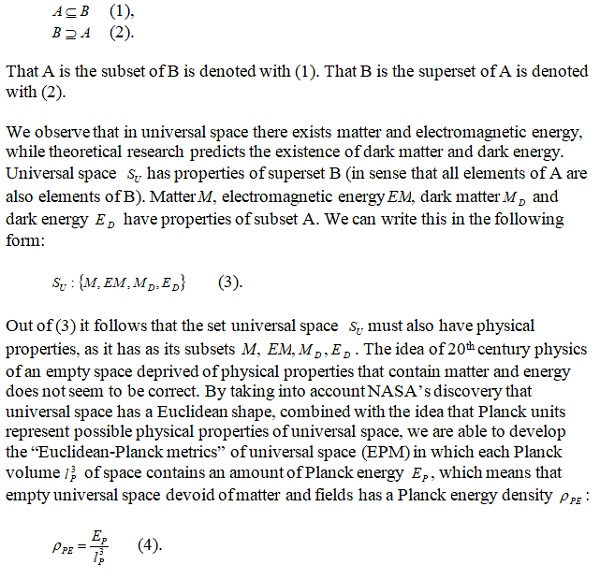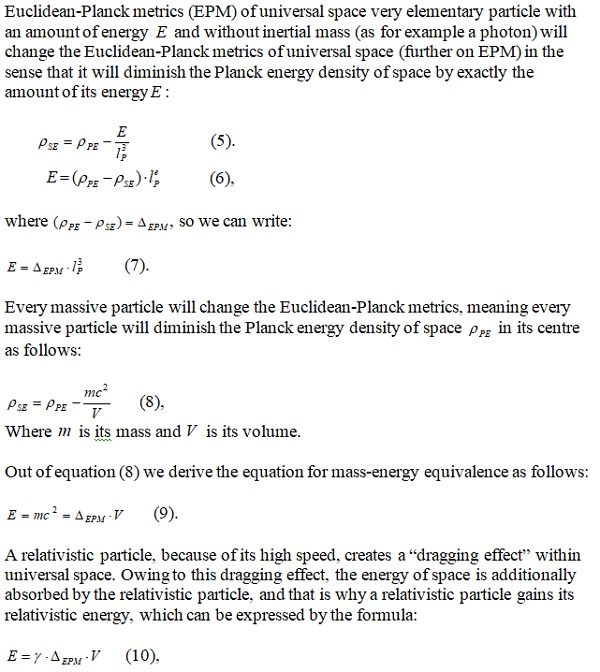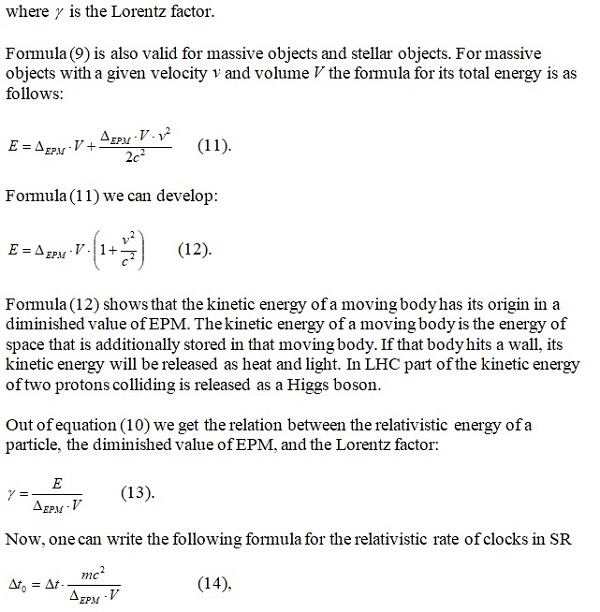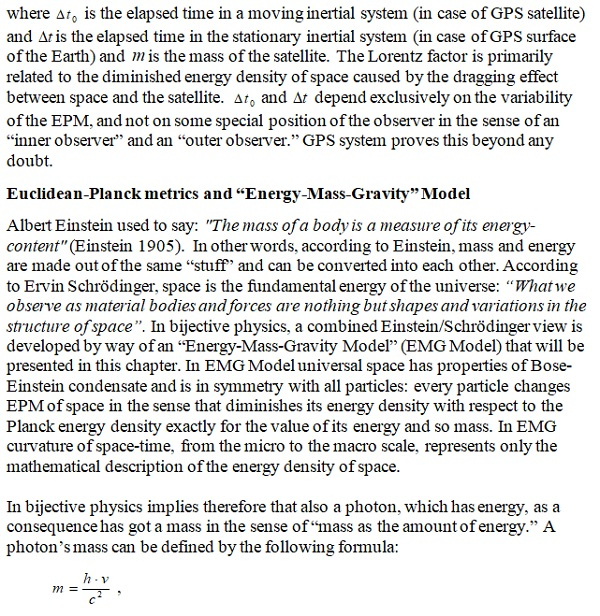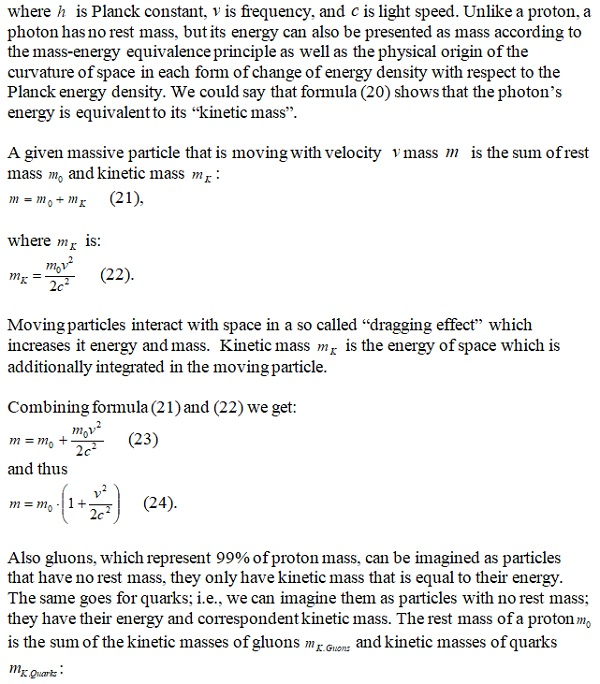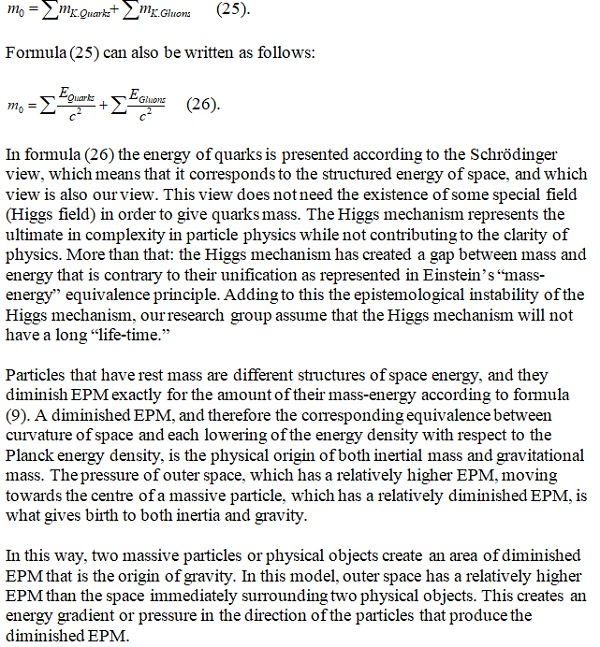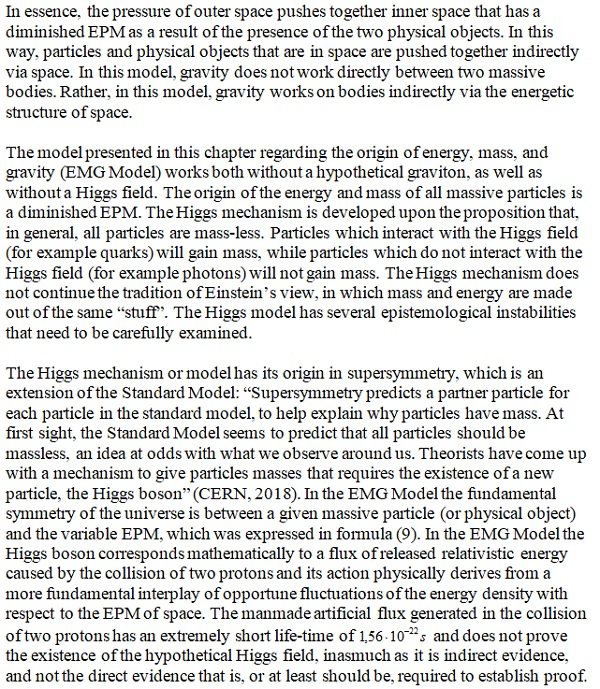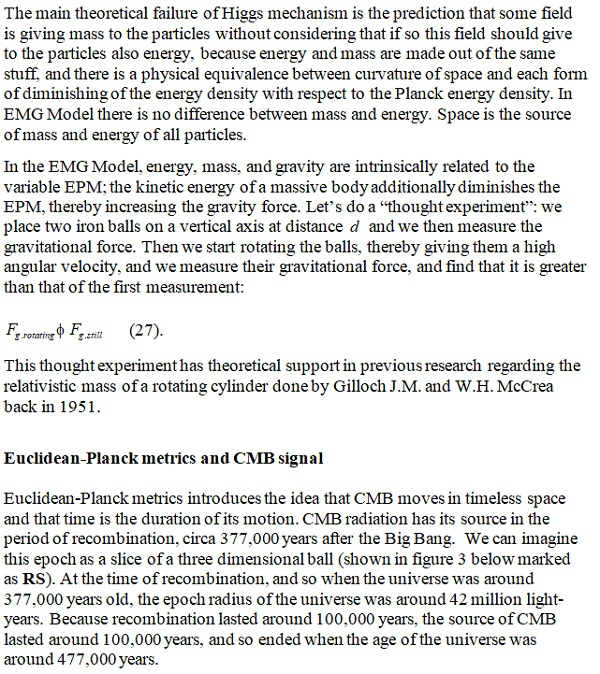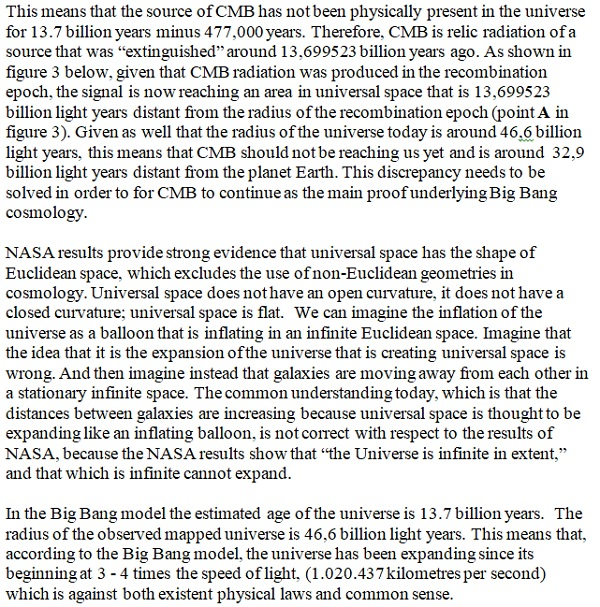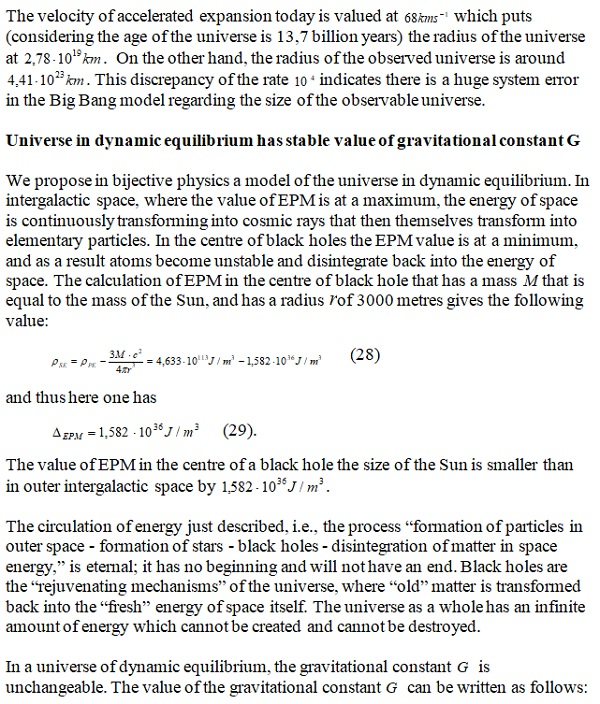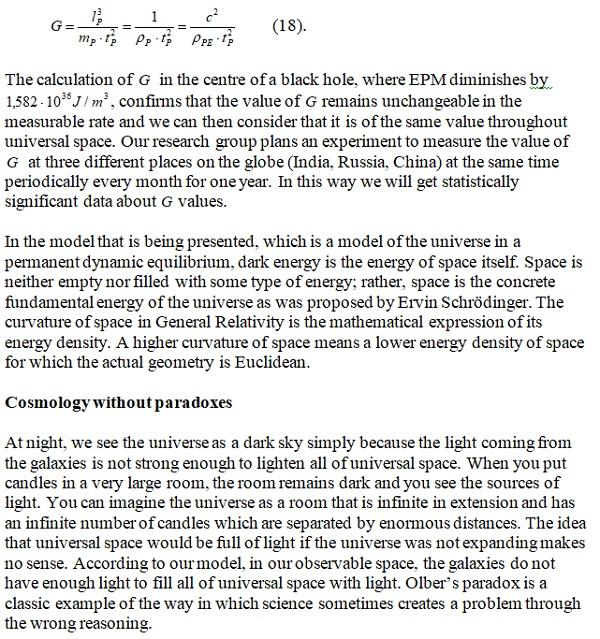The other problem with today’s cosmology is the inflation model, which unsuccessfully tries to explain the appearance of energy in the first moments of the Big Bang. There is no reasonable explanation for the appearance of energy in the so-called “first moments” of the Big Bang. We have known in physics, since the time of Newton, that energy cannot be created and cannot be destroyed; but it can be transformed. The Big Bang model does not satisfy the law of conservation of energy. On the other hand, the model of the universe presented here, which describes the universe as being in dynamic equilibrium, fully satisfies the law of energy conservation.

The inflation signal could not be detected by BICEP2 because it could not have yet reached the Earth. In the cosmological model that we present in this article, the appearance of energy is not a problem. Another problem with Big Bang cosmology is in its failure to account for both where the energy comes from for the “the initial kick” for the explosion, as well as what exactly it is that has exploded. Our model has no such problems. Big Bang cosmology needs a “creator,” someone who has given an initial energy that causes the “birth” of the universe. In this sense, the Big Bang cosmology has some “biblical elements” that are not deserving of being a part of cosmology. In our model, the universe is a non-created system in a permanent dynamic equilibrium; it works perfectly without a creator.

The idea that particles and fields exist in an empty space deprived of physical properties is the main obstacle standing in the way of the progress of physics. With the introduction of Euclidean-Planck metrics (EPM) of universal space, particle physics gains a new model regarding the origin of energy, mass, and the gravity of elementary particles. More than this, EPM applied to cosmology shows that the Big Bang model has insufficiencies that are unsolvable. NASA results confirm that universal space is Euclidean, which excludes the possibility that universal space could be finite, which itself then excludes the possibility that it could expand. The model of infinite universal space governed by the EPM presented in this article heralds the end of the Big Bang model of cosmology and introduces a model of the universe that is in a permanent dynamic equilibrium.

To be Continued

30-Mar-2019

More by :  Amrit Sorli

Name *

Email ID

Comment *

Characters
Verification Code*